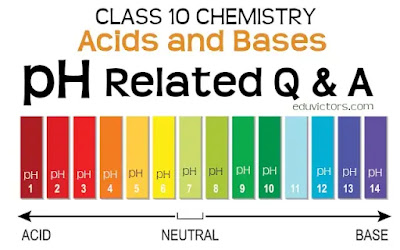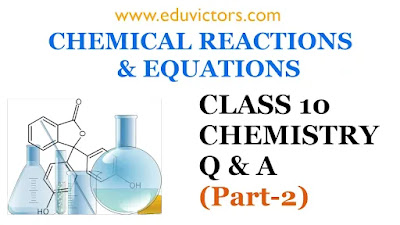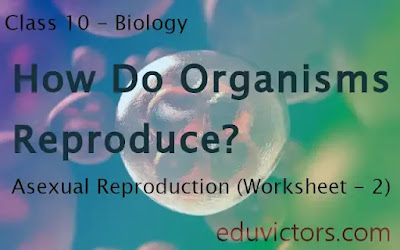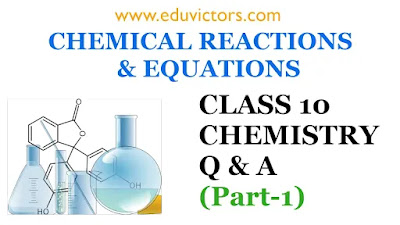## Pages

Showing posts with label class10-science. Show all posts
Showing posts with label class10-science. Show all posts

# Magnetic Effect of Electric Current - MCQs-2

CBSE Class 10 Physics

Buy Oswal Science Question Bank (For 2021 Examination)

Directions: Read the question carefully and choose the right option from the choices given.

Q1: The south pole of a freely suspended magnet points to the:

(a) Geographical south
(b) Geographical north
(c) Slightly left of geographical north
(d) None of these

Q2: For the current-carrying solenoid as shown below, at which point the field strength is maximum?

(a) At point A
(b) At point B
(c) At point C
(d) All of these# CBSE Class 10 Chemistry - Chapter 1: Chemical Reactions and Equations (Part-3)(Q & A)

The first chapter of Chemistry talks about chemical reactions and equations. In a chemical reaction, reactants are transformed into products. In this series, the part-3 video discusses types of chemical reactions.

Following questions are covered:

Q1: What are the important types of chemical reactions.

Q2: What are combination reactions? Give examples.

Q3: Hydrogen burns in oxygen to form water. Identify the type of reaction.# pH related Questions and Answers

CBSE Class 10 - Chemistry

Chapter: Acids and Bases

Q1: How is the strength of acid and base can be estimated?

Answer: Strength of acid and base can be estimated by using universal indicator.

Q2: What is a universal indicator?

Answer: Universal indicator is used to estimate the strength of an acid or a base. It is a mixture of several indicators. It shows different colours at different concentrations of H⁺ ions in the solution.

Q3: What is pH value of a solution?

Answer: The pH of a solution is inversely proportional to the concentration of hydrogen ions in it. Mathematically the pH of a solution is inversely proportional to the concentration of hydrogen ions in it. pH is a pure number, it has no units.# Carbon and Its Compounds - Very Short Answer Based Questions (VSQA)

Class 10 - Chemistry

Very Short Answer Based Questions (VSQA)

Q1: Define catenation.

Answer: The property of a carbon atom to link with other carbon atoms is known as catenation.

Q2: What is isomerism? .

Answer: Isomerism is a phenomenon in which compounds have the same molecular formula but different structural formula. ;

Q3: What are hydrocarbons?

Answer: The compounds containing carbon and hydrogen are known as hydrocarbons. For example, methane (CH₄).# Light (Reflection and Refraction) - Worksheet

CBSE Class 10 - Physics

Directions: Give one-word answer to the following questions (Q1 - Q10).

Q1:  Which property of light changes when it travels from one medium to another?

Q2: Name the ray of light that falls on a mirror.

Q3: How is focal length, (f) of a mirror related to its radius of curvature (R)?

Q4: What kind of beam of light comes out of a car headlight?

Q5: Which mirror forms an image with the following characteristics.
(a) Virtual (b) Erect (c) Same size as the object (d) Laterally inverted# Class 10 - Chemistry - Chemical Reactions and Equations (Q & A) Part-2

The first chapter of Chemistry talks about chemical reactions and equations. In a chemical reaction, reactants are transformed into products. In this series, the part-2 video covers questions and answers about the definition of chemical equation. Understand the difference between unbalanced and balanced chemical equations. Why balancing of chemical equation is necessary? Steps involved in balancing a chemical equation.## Class 10 - Biology - Chapter - How do Organisms Reproduce - Asexual Reproduction (Worksheet - 2)

Fill in the blanks with suitable words:

1. The production of new offspring from a single parent with the involvement of gametes is called ________ reproduction.

2. Reproduction in amoeba takes place asexually called _______   _________.

3. Hydra reproduces asexually, the process is called ________.

4. Leishmania, a unicellular protozoan reproduces very similar to amoeba. But the difference is that the parent cell of Leishmania splits in a _______ plane.## CBSE Class 10 - Chemistry - Chemical Reactions and Equations (Q & A) Part-1

The first chapter of Chemistry talks about chemical reactions and equations. In a chemical reaction, reactants are transformed into products. In this series, the part-1 video covers questions and answers about chemical changes or reactions. How do chemical changes differ from physical changes? Examples of chemical changes and indicators. What are the characteristics or indicators or chemical changes?

For Study Notes, Worksheets, Question Papers and Assignments for CBSE Class 6 - 12 check our website www.eduvictors.com## Control and Coordination (Worksheet - Fill in the Blanks)

CBSE Class 10 - Biology

Fill in the blanks:

1. Control and coordination are the functions of the nervous system and --------------- in our body.

2. The nervous system uses ---------------impulses to transmit messages.

3. Central nervous system consists of -------------and--------------.

4. Largest part of the brain is---------------.

5. The growth of pollen tubes towards the ovules is the result of a ------------- movement.

6. The chemical substances which bring about control and coordination in plants ---------.## Understanding Chemical Changes

CHEMICAL REACTIONS AND EQUATIONS (Q & A)
CBSE Class 10 - Chemistry

Q1: What is a chemical change?

Answer: A chemical change is one in which a new substance is formed which has properties totally different from the starting materials. The starting materials are called as reactants and the new substances formed are called as products. In such changes, the nature and identity of the products is completely changed.

Q2: Give three examples of chemical change?## Friday, 10 April 2020

Most of the subjects covered under CBSE syllabus, NCERT books are recommended. These books have been an essential part of the CBSE curriculum.## CBSE Class 10 Pre-Board Science Sample Question Paper Set-5 (2019-20)

Sample Question Papers for Class 10 are taken into consideration as the best option to understand the question paper pattern very well.

Eduvictors provide collection of sample question papers prepared by various schools and institutes
that help you to practice and get familiar with the question paper pattern. Solving an ample variety of sample papers, together with the last years' pattern papers, has been the recommendation to students.

Sample Question Papers for Science are:

1. Oswaal CBSE Question Bank Class 10 Science Book Chapterwise & Topicwise Includes Objective Types & MCQ's (For March 2020 Exam)

2. Super 10 CBSE Class 10 Science 2020 Exam Sample Papers 2nd Edition

Here follows the Science Sample question papers:## CBSE Class 10 - Science Quiz (2020)

Q1: The shiny finish of wall after white wash is because of

(a) CaO
(b) Ca(OH)₂
(c) CaCO₃
(d) Ca₃(PO₄)₂

Q2: Chemical formula for baking soda is:

(a) NaHCO₃
(b) Na₂CO₃
(c) CaCO₃
(d) Mg(OH)₂

Q3: Green colour coating on copper utensils due to atmospheric corrosion is due to the formation of## CBSE Class 10 - Physics - Study Notes - MCQs, Worksheets, NCERT Solutions

Physics is a pivotal subject for students who wish to seek a career in engineering and other related fields. The majority of the ideas in class 10 Physics are significant as they build the foundations for senior-level physics that are taught in class eleventh and twelfth. Along these lines, having an intensive comprehension of the considerable number of ideas is pivotal to get higher marks in school tests as well as get acquainted with wide a range of information.

In this way, to assist the students with learning the subject more viably, physics notes for class 10 students are given here. These notes based on CBSE syllabus are all around organized, straightforward and spread all the significant points that students need to learn for the tests.

In addition, students can utilize the notes to not just ace in their assessment tests but are effecting in learning the important concepts. There are also 1 mark questions, MCQs and worksheets that will help students evaluate their knowledge about various topics. The notes are written in straightforward language and help students grasp basic concepts easily.

You can check out links to the study notes on various topics, exercises and chapters related to class 10 Physics are given below:## Science - MCQs

CBSE Class 10

Q1: Optical density of a medium depends on

(a) Density of the medium
(b) Thickness of the medium
(c) Velocity of light in that medium
(d) None of these

Q2: Which of the following is not a physical change?

(a) Boiling of water to give water vapour
(b) Melting of ice to give water
(c) Dissolution of salt in water
(d) Combustion of Liquefied Petroleum Gas (LPG)

Q3: Which of the following is correct about chromosomes?

(a) It is composed of DNA and lipids.
(b) It is composed of DNA and proteins.
(c) It is composed of DNA only.
(d) It is composed of RNA and proteins.## CBSE Class 10 Pre-Board Science Sample Question Paper (2019-20)

Sample Question Papers for Class 10 are taken into consideration as the best option to understand the question paper pattern very well.

Eduvictors provide collection of sample question papers prepared by various schools and institutes
that help you to practice and get familiar with the question paper pattern. Solving an ample variety of sample papers, together with the last years' pattern papers, has been the recommendation to students.

Sample Question Papers for Science are:

1. Oswaal CBSE Question Bank Class 10 Science Book Chapterwise & Topicwise Includes Objective Types & MCQ's (For March 2020 Exam)

2. Super 10 CBSE Class 10 Science 2020 Exam Sample Papers 2nd Edition

Here follows the Science Sample question papers:## CBSE Class 10 - Chemistry - Assertion Reason Based Questions

Assertion and Reasoning based questions are bit tricky. You should be able to distinguish whether the assertion is well connected to the reason or these are just random facts. Read both statements carefully, and check if both statements are factually or logically correct or not. If both are correct, then try to check if both are well connected to each other or not.

The questions below consist of statements of an Assertion and a Reason. Use the following key to choose appropriate answer:

(A) If both assertion and reason are CORRECT and reason is the CORRECT explanation of the assertion.
(B) If both assertion and reason are CORRECT, but reason is NOT THE CORRECT explanation of the assertion.
(C) If assertion is CORRECT, but reason is INCORRECT
(D) If assertion is INCORRECT, but reason is CORRECT

QUESTIONS:

1.  ASSERTION:  Combustion reactions are also called exothermic oxidation reactions.
REASON:  In these reactions, oxygen is added and heat energy is released.## CBSE Class 10 Pre-Board Science Sample Question Paper (2019-20)

Sample Question Papers for Class 10 are taken into consideration as the best option to understand the question paper pattern very well.

Eduvictors provide collection of sample question papers prepared by various schools and institutes
that help you to practice and get familiar with the question paper pattern. Solving an ample variety of sample papers, together with the last years' pattern papers, has been the recommendation to students.

Sample Question Papers for Science are:

1. Oswaal CBSE Question Bank Class 10 Science Book Chapterwise & Topicwise Includes Objective Types & MCQ's (For March 2020 Exam)

2. Super 10 CBSE Class 10 Science 2020 Exam Sample Papers 2nd Edition

Here follows the Science Sample question papers:## CBSE Class 10 Pre-Board Science Sample Question Paper (2019-20)

Sample Question Papers for Class 10 are taken into consideration as the best option to understand the question paper pattern very well.

Eduvictors provide collection of sample question papers prepared by various schools and institutes
that help you to practice and get familiar with the question paper pattern. Solving an ample variety of sample papers, together with the last years' pattern papers, has been the recommendation to students.

Sample Question Papers for Science are:

1. Oswaal CBSE Question Bank Class 10 Science Book Chapterwise & Topicwise Includes Objective Types & MCQ's (For March 2020 Exam)

2. Super 10 CBSE Class 10 Science 2020 Exam Sample Papers 2nd Edition

Here follows the Science Sample question papers:## CBSE Class 10 Science Sample Question Papers (2019-20)

Sample Question Papers for Class 10 are taken into consideration as the best option to understand the question paper pattern very well.

Eduvictors provide collection of sample question papers prepared by various schools and institutes
that help you to practice and get familiar with the question paper pattern. Solving an ample variety of sample papers, together with the last years' pattern papers, has been the recommendation to students.

Sample Question Papers for Science are:

1. Oswaal CBSE Question Bank Class 10 Science Book Chapterwise & Topicwise Includes Objective Types & MCQ's (For March 2020 Exam)

2. Super 10 CBSE Class 10 Science 2020 Exam Sample Papers 2nd Edition

Here follows the Science Sample question papers: